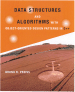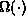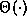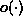Data Structures and Algorithms with Object-Oriented Design Patterns in C++## Outline

This book presents material identified in the Computing Curricula 1991 report of the ACM/IEEE-CS Joint Curriculum Task Force. The book specifically addresses the following knowledge units: AL1: Basic Data structures, AL2: Abstract Data Types, AL3: Recursive Algorithms, AL4: Complexity Analysis, AL6: Sorting and Searching, and AL8: Problem-Solving Strategies. The breadth and depth of coverage is typical of what should appear in the second or third year of an undergraduate program in computer science/computer engineering.

In order to analyze a program, it is necessary to develop a model of the computer. Chapterdevelops several models and illustrates with examples how these models predict performance. Both average-case and worst-case analyses of running time are considered. Recursive algorithms are discussed and it is shown how to solve a recurrence using repeated substitution. This chapter also reviews arithmetic and geometric series summations, Horner's rule and the properties of harmonic numbers.

Chapterintroduces asymptotic (big-oh) notation and shows by comparing with Chapterthat the results of asymptotic analysis are consistent with models of higher fidelity. In addition to, this chapter also covers other asymptotic notations (,and) and develops the asymptotic properties of polynomials and logarithms.

Chapterintroduces the foundational data structures--the array and the linked list. Virtually all the data structures in the rest of the book can be implemented using either one of these foundational structures. This chapter also covers multi-dimensional arrays and matrices.

Chapterdeals with abstraction and data types. It presents the recurring design patterns used throughout the text as well a unifying framework for the data structures presented in the subsequent chapters. In particular, all of the data structures are viewed as abstract containers.

Chapterdiscusses stacks, queues and deques. This chapter presents implementations based on both foundational data structures (arrays and linked lists). Applications for stacks and queues and queues are presented.

Chaptercovers ordered lists, but sorted and unsorted. In this chapter, a list is viewed as a searchable container. Again several applications of lists are presented.

Chapterintroduces hashing and the notion of a hash table. This chapter addresses the design of hashing functions for the various basic data types as well as for the abstract data types described in Chapter. Both scatter tables and hash tables are covered in depth and analytical performance results are derived.

Chapterintroduces trees and describes their many forms. Both depth-first and breadth-first tree traversals are presented. Completely generic traversal algorithms based on the use of the visitor design pattern are presented, thereby illustrating the power of algorithmic abstraction. This chapter also shows how trees are used to represent mathematical expressions and illustrates the relationships between traversals and the various expression notations (prefix, infix and postfix).

Chapteraddresses trees as searchable containers. Again, the power of algorithmic abstraction is demonstrated by showing the relationships between simple algorithms and balancing algorithms. This chapter also presents average case performance analyses and illustrates the solution of recurrences by telescoping.

Chapterpresents several priority queue implementations, including binary heaps, leftist heaps and binomial queues. In particular this chapter illustrates how a more complicated data structure (leftist heap) extends an existing one (tree). Discrete-event simulation is presented as an application of priority queues.

Chaptercovers sets and multisets. Also covered are partitions and disjoint set algorithms. The latter topic illustrates again the use of algorithmic abstraction.

Techniques for dynamic storage management are presented in Chapter. This is a topic that is not found often in texts of this sort. However, the features of C++ which allow the user to redefine the new and delete operators make this topic approachable.

Chaptersurveys a number of algorithm design techniques. Included are brute-force and greedy algorithms, backtracking algorithms (including branch-and-bound), divide-and-conquer algorithms and dynamic programming. An object-oriented approach based on the notion of an abstract solution space and an abstract solver unifies much of the discussion. This chapter also covers briefly random number generators, Monte Carlo methods, and simulated annealing.

Chaptercovers the major sorting algorithms in an object-oriented style based on the notion of an abstract sorter. Using the abstract sorter illustrates the relationships between the various classes of sorting algorithm and demonstrates the use of algorithmic abstractions.

Finally, Chapterpresents an overview of graphs and graph algorithms. Both depth-first and breadth-first graph traversals are presented. Topological sort is viewed as yet another special kind of traversal. Generic traversal algorithms based on the visitor design pattern are presented, once more illustrating algorithmic abstraction. This chapter also covers various shortest path algorithms and minimum-spanning-tree algorithms.

At the end of each chapter is a set of exercises and a set of programming projects. The exercises are designed to consolidate the concepts presented in the text. The programming projects generally require the student to extend the implementation given in the text.Copyright © 1997 by Bruno R. Preiss, P.Eng. All rights reserved.